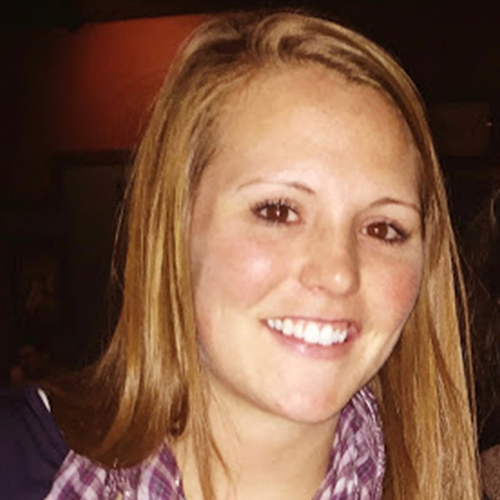• 1
Grade 1
• Measurement
• 1.1
Metric Units of Length
Taught by• 1.2
Customary Units of Length with 3 Numbers
Taught by• 1.3
Metric Units of Length: 3 Numbers
Taught by• 1.4
Customary Units of Length
Taught by• 1.87
Length and Height
Taught by• 1.88
Which Customary Unit of Length Is Appropriate?
Taught by• 1.89
Which Metric Unit of Length Is Appropriate?
Taught by• Time
• 1.5
Choose the Appropriate Time Units
Taught by• 1.90
Reading Clocks
Taught by• 1.91
Match Clocks and Time
Taught by• 1.92
Match Analog and Digital Clocks
Taught by• 1.93
Compare Clocks
Taught by• Counting
• 1.6
Fewer and More - Comparing Groups of Mixed Objects
Taught by• 1.7
Count Groups of Tens and Ones Up to 20
Taught by• 1.8
Count Groups of Ten to 20, 30, 120
Taught by• 1.40
Counting and Number Patterns: Writing Numbers in Words
Taught by• 1.41
Counting and Number Patterns: Hundred Chart
Taught by• 1.49
Counting Forward and Backward
Taught by• 1.52
Count Groups of Tens and Ones Up to 100
Taught by• 1.64
Count Objects Up to 120
Taught by• 1.65
Count Objects Up to 20
Taught by• 1.67
Skip Counting by 5, 10 and 100
Taught by• 1.68
Counting and Number Patterns: Skip-Counting Sequences
Taught by• 1.69
Count by a Specific Number
Taught by• Addition
• 1.8
Count Groups of Ten to 20, 30, 120
Taught by• 1.9
Add Tens
Taught by• 1.10
Add Two Numbers Up to 100
Taught by• 1.11
Adding Tens and Ones
Taught by• 1.12
Addition with Sums Up to 18
Taught by• 1.13
Addition Sentences Up to 20
Taught by• 1.14
Complete the Equation with Sums Up to 20
Taught by• 1.15
Add and Subtract Numbers Up to 18
Taught by• 1.16
Add Three Numbers Up to 20
Taught by• 1.17
3 Numbers
Taught by• 1.18
Related Addition Equations Up to 10
Taught by• 1.19
How to Make a Number with Single Digits Up to 10
Taught by• 1.20
Addition with a Specific Number
Taught by• 1.21
Add Two Numbers Up to 20
Taught by• 1.22
Adding Doubles
Taught by• 1.34
Addition with Pictures Up to 10, 20
Taught by• 1.35
Choose Addition Pictures Up to 10
Taught by• Number Properties
• 1.18
Related Addition Equations Up to 10
Taught by• 1.19
How to Make a Number with Single Digits Up to 10
Taught by• 1.27
Related Subtraction Equations Up to 10
Taught by• 1.28
Ways to Make a Number Up to 18
Taught by• 1.49
Counting Forward and Backward
Taught by• 1.51
Put Numbers in Order Up to 120
Taught by• Mixed Equations
• 1.23
Ten More or Less
Taught by• 1.48
Add and Subtract Tens
Taught by• 1.50
Which Sign Makes the Number Sentence True?
Taught by• 1.56
Add and Subtract - How to Make a Number
Taught by• 1.70
Addition and Subtraction Up to 20
Taught by• Subtraction
• 1.24
Subtract Tens
Taught by• 1.25
Subtraction Sentences with Numbers Up to 18
Taught by• 1.26
Complete the Subtraction Sentence with Numbers Up to 18
Taught by• 1.27
Related Subtraction Equations Up to 10
Taught by• 1.28
Ways to Make a Number Up to 18
Taught by• 1.29
Subtracting Zero and All
Taught by• 1.30
Subtracting Doubles
Taught by• 1.31
Subtract Two Numbers Up to 18
Taught by• 1.32
Subtract One-Digit Numbers from Two-Digit Numbers
Taught by• 1.33
Subtraction with a Specific Number
Taught by• 1.37
Subtraction with Pictures Up to 10
Taught by• 1.38
Choose Subtraction Pictures with Numbers Up to 10
Taught by• Fractions
• 1.36
What Fraction Does This Shape Show?
Taught by• 1.47
Equal Parts
Taught by• 1.84
Identify Fractions of Shapes
Taught by• 1.85
Which Shape Matches the Fraction?
Taught by• 1.86
Compare Fractions - Same Numerator or Denominator
Taught by• Graphing
• 1.39
Venn Diagrams
Taught by• 1.43
Interpret Tally Charts, Picture Graphs and Tables
Taught by• 1.44
Interpret Bar Graphs
Taught by• 1.45
Which Bar Graph Is Correct?
Taught by• Comparison
• 1.39
Venn Diagrams
Taught by• 1.45
Which Bar Graph Is Correct?
Taught by• 1.51
Put Numbers in Order Up to 120
Taught by• Number Patterns
• 1.41
Counting and Number Patterns: Hundred Chart
Taught by• 1.68
Counting and Number Patterns: Skip-Counting Sequences
Taught by• 1.69
Count by a Specific Number
Taught by• Money
• 1.57
Names and Values of Money I
Taught by• 1.58
Names and Values of Money II
Taught by• 1.59
Count Money
Taught by• 1.60
Equivalent Amounts of Money
Taught by• 1.61
Equivalent Coins I
Taught by• 1.62
Equivalent Coins II
Taught by• 1.63
Least Number of Coins
Taught by• Geometry
• 1.71
Identify Shapes I
Taught by• 1.72
Identify Shapes II
Taught by• 1.75
Compare Sides and Corners
Taught by• 1.76
Count Sides and Corners
Taught by• 1.77
Count Edges, Vertices and Faces
Taught by• 1.78
Geometry of Everyday Objects
Taught by• 1.79
Identify Solid Figures
Taught by• 1.80
Relate Planar and Solid Figures
Taught by• 1.81
Count Edges
Taught by• 1.82
Count Vertices
Taught by• 1.83
Count Faces
Taught byGet a step-by-step tutorial from math teachers that go over all the math skills in the first grade. As math skills become more complicated, sometimes students need to go over lessons again even after school is done. On-demand videos are available for students who are stuck or just need a refresher on a math lesson.

• Math teachers go over their best strategies specifically designed for young students who are learning the foundations of math.
• Teachers show students how to use the scratchpad to take notes and keep track of the numbers.
• Students get the chance to go over math skills for positions, counting, graphing, and many more.